# How to calculate the interference voltage?

#### Jiwoo

Joined Feb 6, 2023
19
Hi,

I need to calculate the inteference voltage. The task is described as following: "LB1 and LB1 run parallel. The coupling capacitance between LB1 and LB2 is 10pF. At time t=0 the switch S is thrown. Calculate the general interference voltage uv resulting from the capacitive coupling via the parallel printed conductors LB1 and LB2. It applies Uc(t=0) = 10V." Messverstärker means measurement amplifier.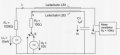Could you somehow help me to draw the simplified equivalent circuit to have a better overview? Because I can't tell where the inteference voltage uv drops. Thanks for the help

#### Jiwoo

Joined Feb 6, 2023
19
@ericgibbs Hi, thanks for the answer. I have 2 questions tho: 1. What is R3 and how did you determine it?
2. What about the inductance LB1 and LB2? They should be up there as well right?

#### ericgibbs

Joined Jan 29, 2010
17,423
hi J,
The Measurement amplifier has an Input Impedance of 10Meg Ohms, [refer to your posted image] so that is the load for the interference signal.

I don't see any Inductance values quoted for LB1 and LB2.?

The LB1 and LB2 refer to the actual Printed Circuit Boards under test, parallel printed conductors LB1 and LB2.

I would like to see your final calculations for the actual signal value.
E
translate image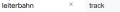Last edited:

#### Jiwoo

Joined Feb 6, 2023
19
@ericgibbs Oh ok, then it was just a little mistake I guess, cuz your circuit says "1Meg" instead of 10MegOh nvm. there are no inductance values quoted.

Well I guess uv equals uc1 + uc2. uc1 is 1uF. Regarding uc2, I am not sure how to calculate there the voltage. The voltage on R2 must be U2 - uc. Thorugh which way can I determine uc2?

#### ericgibbs

Joined Jan 29, 2010
17,423
hi J,
Consider the initial state
The C1 capacitor is not charged, U1 is 10mV across the 10meg load via R1=100k
so I1 current is U1/(R1+RE)

I2 current is Zero

Switch S closes and U2 charges the C1=1uF capacitor via the R2=100R resistor,,,,,, you work out the exponential voltage charge on C1.
So I2 current will also be an exponential current.
...
Assume the capacitor charges to U2 in five Time Constants, then S switch is closed to 0V line, so that C1 discharges via R2 to zero, again assume 5 time constants to discharge to zero.

Do you know how to make these calculations?

Added and image to show the C1 charge state [ I have hidden the Vertical voltage scale on the plot]

E

Added summary, when you understand how the circuit works, answer the original question
This time assume that C1 is fully charged to 10V then S switch is closed in order to discharge C1
via R2.
Remember, this discharge current induces a voltage into LB1 via the stated 10pF coupling between LB1 and LB2.
This is the Voltage you have been asked to calculate.

E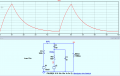Last edited:

#### ericgibbs

Joined Jan 29, 2010
17,423
hi J
It may be easier to consider the original question, assuming that C1 is fully charged to 10V.
Then calculate the discharge time through the 100 Ohm resistor, when switch S is Closed.

Can you calculate v= V exp-(t/RC)

E

#### ericgibbs

Joined Jan 29, 2010
17,423
hi J,
When you have that discharge value, the next step is to apply that discharge voltage across the 10p and the 100k in Series.
As the measuring unit has an input resistance of 10Meg I would ignore its effect of being in parallel with the 100k.

Knowing the above values, you can calculate the current through the Series circuit, and the voltage across the 100k, don't forget to correct for the U1 10mV.

E

#### ericgibbs

Joined Jan 29, 2010
17,423
hi J,
As you have shown, the time constant of one,100uSec from 10V down 3.68V.
This exponential waveform is applied across the 10pF and the 100k in series, so the current through these two components can be calculated.
Adjust the current to allow for the U1, 10mV source, knowing this current you can calculate the required uv voltage

E
This image shows the input waveform you have calculated.

BTW: remember the original question.
Calculate the general interference voltage uv resulting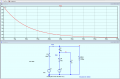is

Last edited:
•Jiwoo

#### Jiwoo

Joined Feb 6, 2023
19
@ericgibbs But is it necessery to use complex numbers? Because I have the impedance of C2 :/ What do you mean by "adjust the current to allow U1"?

#### ericgibbs

Joined Jan 29, 2010
17,423
hi @Jiwoo
When the switch is closed C1 discharges through R2, this makes vc 10v negative with respect to uv, so the 10p charges almost instantly to ~10v, set by the time constant of C2 and R1.
10^-11* 10^5 = 10^-6, ie 1uSec

This initial ~1uS C2 charge current flows through the R1 resistor, which causes a voltage drop across R1, which is measured as uv peak.
Then the voltage across C2 follows the discharge profile of the R2/C1 discharge.

You don’t need complex numbers for general interference voltage
Look at this plot for guidance.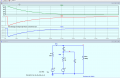Last edited:

#### Jiwoo

Joined Feb 6, 2023
19
@ericgibbs But the current would be uc(t)/(R1+jwc2) or am I missing something? :/

#### ericgibbs

Joined Jan 29, 2010
17,423
Hi @Jiwoo
As this a college assignment, I can only give guidance.

Look at the top row images, they show as an example what happens when the +10V U2 is removed instantly.
Vc falls to Zero, which means the voltage across the 10p is now –10V, making V(uv) equal to –10V. So the 10p discharges via R1 100k, in approx 1uSec to one time constant.
The lower row images show the full circuit, when C1 is connected to 0v via the S switch and R2 100R, C1 will discharge with time constant C1 & R2 ~ 100uSec.
Looking at the V(uv) plot you see a sharp drop from +10mV [U1] in approx 1uSec due to the TC for C2 and R1.
BUT
Vc voltage continues to fall exponentially with a TC of 100uSec, and the voltage across the 10p also reduces at the same rate, so the V(uv) voltage tracks the Vc voltage and so the V(uv) voltage falls at the same rate from its initial value of approx –90mV.

It is all about Time constants of C1/R2 and C2/R1, no complex.
E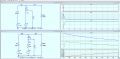•Jiwoo

#### Jiwoo

Joined Feb 6, 2023
19
@ericgibbs I understand the procedure, but the problem is I dont know how to put these information in a formula to calculate what I want! I have no Idea how to determine the current through the time constants. I only know the formula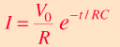which means I need C2 instead of R as a denominator. The only thing that goes through my mind is to try Uv = 10mV *e^(-t/R1*C2). I don't know further I am sorry.

#### ericgibbs

Joined Jan 29, 2010
17,423
hi @Jiwoo
Lets try this,
Assume you have 10pF charged to 10V, then you switch a 100k across the capacitor, what will be the instantaneous current through the resistor.
And what will be the current at one time constant.
E

•Jiwoo